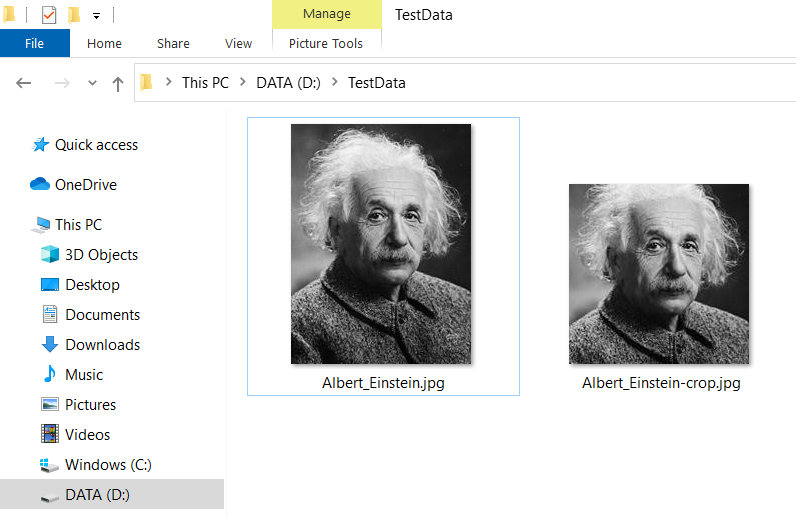In this tutorial we learn how to crop an image file to square using the Scalr class of Imgscalr library.

#### How to add Imgscalr library to the Java project

To use the Imgscalr library in the Gradle build project, add the following dependency into the build.gradle file.

``implementation 'org.imgscalr:imgscalr-lib:4.2'``

To use the Imgscalr library in the Maven build project, add the following dependency into the pom.xml file.

``````<dependency>
<groupId>org.imgscalr</groupId>
<artifactId>imgscalr-lib</artifactId>
<version>4.2</version>
</dependency>``````

#### How to crop an image to square in Java

The Imgscalr library provides the method Scalr.crop() to allow cropping images. In the following Java example code we show you how to use the Scalr.crop() method to crop a given image to square size.

CropImageToSquareExample.java

``````import org.imgscalr.Scalr;

import javax.imageio.ImageIO;
import java.awt.image.BufferedImage;
import java.io.File;
import java.io.IOException;

public class CropImageToSquareExample {
public static void main(String... args) {
String originalFilePath = "D:\\TestData\\Albert_Einstein.jpg";
String targetFilePath = "D:\\TestData\\Albert_Einstein-crop.jpg";

cropImageToSquare(originalFilePath, targetFilePath);
}

public static void cropImageToSquare(String originalFilePath, String scopedFilePath) {
try {
File sourceFile = new File(originalFilePath);
int width = originalImage.getWidth();
int height = originalImage.getHeight();
int x, y, cropWidth, cropHeight;
if(width > height) {
x = (width - height) / 2;
y = 0;
cropWidth = cropHeight = height;
} else {
x = 0;
y = (height - width) / 2;
cropWidth = cropHeight = width;
}

BufferedImage resizedImage = Scalr.crop(originalImage, x, y, cropWidth, cropHeight);

File resizedFile = new File(scopedFilePath);
ImageIO.write(resizedImage, "jpg", resizedFile);

originalImage.flush();
resizedImage.flush();
} catch (IOException e) {
e.printStackTrace();
}
}
}``````
The output is:Happy Coding 😊